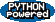# Progress measures

How can one prove properties about infinite limitations by looking at the finite ones? To study this question, I formulated in my thesis (Progress measures and finite arguments for infinite computations, Cornell University, 1990) ways of viewing infinite limitations as limits of finite ones. In particular, I showed how one can directly quantify progress towards membership in various sets of the Borel hierarchy through progress measures, which are arrangements of ordinals. After my thesis, I applied these techniques to show that certain tree automata can be complemented in exponential space, instead of doubly-exponential space; this result means that the lower bound, established by Meyer, for the logic S2S of an one exponential increase in complexity per quantifer alternation is also an upper bound. The progress measures also summarize in one mathematical entity earlier program transformation techniques for reasoning about termination under fairness conditions.

For some publications in this area, see progress measure publications.

 XSLT &